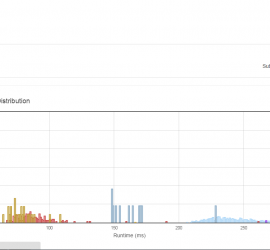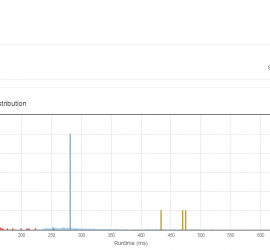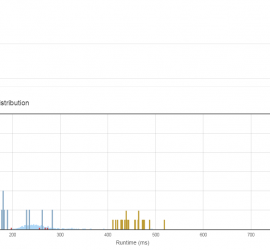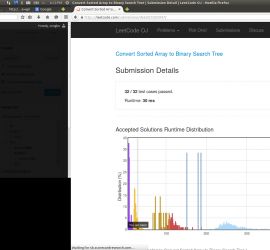# Daily Archives: April 8, 2015

## [leetcode] Maximum Subarray

Maximum Subarray Find the contiguous subarray within an array (containing at least one number) which has the largest sum. For example, given the array [−2,1,−3,4,−1,2,1,−5,4], the contiguous subarray [4,−1,2,1] has the largest sum = 6. click to show more practice. More practice:If you have figured out the O(n) solution, try […]## [leetcode] Permutations II

Permutations II Given a collection of numbers that might contain duplicates, return all possible unique permutations. For example, [1,1,2] have the following unique permutations: [1,1,2], [1,2,1], and [2,1,1]. 跟PermutionsI类似，不过需要去重。 思路是先对原数组排序，然后在循环开始时，跳过重复元素。 class Solution { public: vector<vector<int> > permuteUnique(vector<int> &num) { vector<vector<int>> re; vector<int> thisRe; sort(num.begin(), num.end()); DFS(num, re, thisRe); return re; […]## [leetcode] Permutations

Permutations Given a collection of numbers, return all possible permutations. For example, [1,2,3] have the following permutations: [1,2,3], [1,3,2], [2,1,3], [2,3,1], [3,1,2], and [3,2,1]. 深搜DFS。注意参数需要传引用。 class Solution { public: vector<vector<int> > permute(vector<int> &num) { vector<vector<int>> re; vector<int> thisRe; DFS(num, re, thisRe); return re; } void DFS(vector<int> &num, vector<vector<int>> &re, vector<int> […]## [leetcode] Convert Sorted Array to Binary Search Tree

Convert Sorted Array to Binary Search Tree Given an array where elements are sorted in ascending order, convert it to a height balanced BST.a 简单的深搜问题。 每次取mid=(start + end) / 2; 然后以num[mid]为root，深搜root->left, 和root->right。 复杂度o(n) /** * Definition for binary tree * struct TreeNode { * int val; * TreeNode *left; * […]